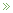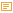• ## The Meaning of the Gaussian Kernel

Posted by Konstantin 11.11.2016

A question on Quora reminded me that I wanted to post this explanation here every time I got a chance to teach SVMs and Kernel methods, but I never found the time. The post expects basic knowledge of those topics from the reader.

### Introductory Background

The concept of kernel methods is probably one of the coolest tricks in machine learning. With most machine learning research nowadays being centered around neural networks, they have gone somewhat out of fashion recently, but I suspect they will strike back one day in some way or another.

The idea of a kernel method starts with the curious observation that if you take a dot product of two vectors,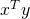, and square it, the result can be regarded as a dot product of two "feature vectors", where the features are all pairwise products of the original inputs: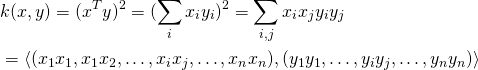Analogously, if you raise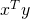to the third power, you are essentially computing a dot product within a space of all possible three-way products of your inputs, and so on, without ever actually having to see those features explicitly.

If you now take any linear model (e.g. linear regression, linear classification, PCA, etc) it turns out you can replace the "real" dot product in its formulation model with such a kernel function, and this will magically convert your model into a linear model with nonlinear features (e.g. pairwise or triple products). As those features are never explicitly computed, there is no problem if there were millions or billions of them.

Consider, for example, plain old linear regression: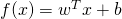. We can "kernelize" it by first representing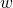as a linear combination of the data points (this is called a dual representation):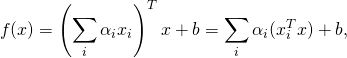and then swapping all the dot products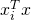with a custom kernel function: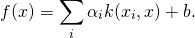If we now substitute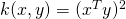here, our model becomes a second degree polynomial regression. If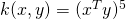it is the fifth degree polynomial regression, etc. It's like magic, you plug in different functions and things just work.

It turns out that there are lots of valid choices for the kernel function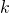, and, of course, the Gaussian function is one of these choices:It is not too surprising - the Gaussian function tends to pop up everywhere, after all, but it is not obvious what "implicit features" it should represent when viewed as a kernel function. Most textbooks do not seem to cover this question in sufficient detail, usually, so let me do it here.

### The Gaussian Kernel

To see the meaning of the Gaussian kernel we need to understand the couple of ways in which any kernel functions can be combined. We saw before that raising a linear kernel to the powermakes a kernel with a feature space, which includes all-wise products. Now let us examine what happens if we add two or more kernel functions. Consider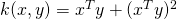, for example. It is not hard to see that it corresponds to an inner product of feature vectors of the form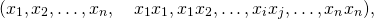i.e. the concatenation of degree-1 (untransformed) features, and degree-2 (pairwise product) features.

Multiplying a kernel function with a constantis also meaningful. It corresponds to scaling the corresponding features by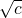. For example,.

Still with me? Great, now let us combine the tricks above and consider the following kernel: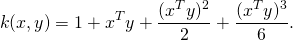Apparently, it is a kernel which corresponds to a feature mapping, which concatenates a constant feature, all original features, all pairwise products scaled down by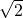and all triple products scaled down by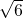.

Looks impressive, right? Let us continue and add more members to this kernel, so that it would contain all four-wise, five-wise, and so on up to infinity-wise products of input features. We shall choose the scaling coefficients for each term carefully, so that the resulting infinite sum would resemble a familiar expression:We can conclude here that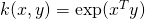is a valid kernel function, which corresponds to a feature space, which includes products of input features of any degree, up to infinity.

But we are not done yet. Suppose that we decide to normalize the inputs before applying our linear model. That is, we want to convert each vectortobefore feeding it to the model. This is quite often a smart idea, which improves generalization. It turns out we can do this “data normalization” without really touching the data points themselves, but by only tuning the kernel instead.

Consider again the linear kernel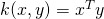. If we normalize the vectors before taking their inner product, we get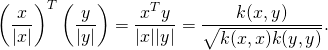With some reflection you will see that the latter expression would normalize the features for any kernel.

Let us see what happens if we apply this kernel normalization to the “infinite polynomial” (i.e. exponential) kernel we just derived: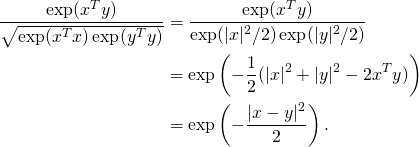Voilà, the Gaussian kernel. Well, it still lacksin the denominator but by now you hopefully see that adding it is equivalent to scaling the inputs by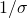To conclude: the Gaussian kernel is a normalized polynomial kernel of infinite degree (where feature products if-th degree are scaled down by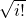before normalization). Simple, right?

### An Example

The derivations above may look somewhat theoretic if not "magical", so let us work through a couple of numeric examples. Suppose our original vectors are one-dimensional (that is, real numbers), and let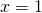,. The value of the Gaussian kernelfor these inputs is: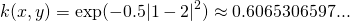Let us see whether we can obtain the same value as a simple dot product of normalized polynomial feature vectors of a high degree. For that, we first need to compute the corresponding unnormalized feature representation: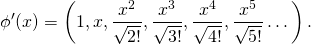As our inputs are rather small in magnitude, we can hope that the feature sequence quickly approaches zero, so we don't really have to work with infinite vectors. Indeed, here is how the feature sequences look like:

----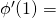(1, 1, 0.707, 0.408, 0.204, 0.091, 0.037, 0.014, 0.005, 0.002, 0.001, 0.000, 0.000, ...)

----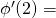(1, 2, 2.828, 3.266, 3.266, 2.921, 2.385, 1.803, 1.275, 0.850, 0.538, 0.324, 0.187, 0.104, 0.055, 0.029, 0.014, 0.007, 0.003, 0.002, 0.001, ...)

Let us limit ourselves to just these first 21 features. To obtain the final Gaussian kernel feature representations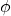we just need to normalize:

----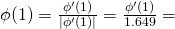(0.607, 0.607, 0.429, 0.248, 0.124, 0.055, 0.023, 0.009, 0.003, 0.001, 0.000, ...)

----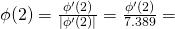(0.135, 0.271, 0.383, 0.442, 0.442, 0.395, 0.323, 0.244, 0.173, 0.115, 0.073, 0.044, 0.025, 0.014, 0.008, 0.004, 0.002, 0.001, 0.000, ...)

Finally, we compute the simple dot product of these two vectors: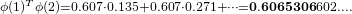In boldface are the decimal digits, which match the value of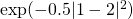. The discrepancy is probably more due to lack of floating-point precision rather than to our approximation.

### A 2D Example

The one-dimensional example might have seemed somewhat too simplistic, so let us also go through a two-dimensional case. Here our unnormalized feature representation is the following: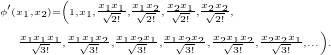This looks pretty heavy, and we didn't even finish writing out the third degree products. If we wanted to continue all the way up to degree 20, we would end up with a vector with 2097151 elements!

Note that many products are repeated, however (e.g.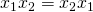), hence these are not really all different features. Let us try to pack them more efficiently. As you'll see in a moment, this will open up a much nicer perspective on the feature vector in general.

Basic combinatorics will tell us, that each feature of the form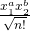must be repeated exactlytimes in our current feature vector. Thus, instead of repeating it, we could replace it with a single feature, scaled by. "Why the square root?" you might ask here. Because when combining a repeated feature we must preserve the overall vector norm. Consider a vector, for example. Its norm is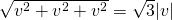, exactly the same as the norm of the single-element vector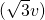.

As we do this scaling, each feature gets converted to a nice symmetric form: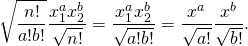This means that we can compute the 2-dimensional feature vector by first expanding each parameter into a vector of powers, like we did in the previous example, and then taking all their pairwise products. This way, if we wanted to limit ourselves with maximum degree 20, we would only have to deal with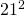= 231 features instead of 2097151. Nice!

Here is a new view of the unnormalized feature vector up to degree 3: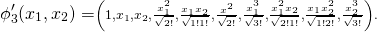Let us limit ourselves to this degree-3 example and let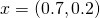,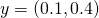(if we picked larger values, we would need to expand our feature vectors to a higher degree to get a reasonable approximation of the Gaussian kernel). Now:

----(1, 0.7, 0.2, 0.346, 0.140, 0.028, 0.140, 0.069, 0.020, 0.003),

----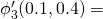(1, 0.1, 0.4, 0.007, 0.040, 0.113, 0.000, 0.003, 0.011, 0.026).

After normalization:

----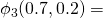(0.768, 0.538, 0.154, 0.266, 0.108, 0.022, 0.108, 0.053, 0.015, 0.003),

----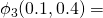(0.919, 0.092, 0.367, 0.006, 0.037, 0.104, 0.000, 0.003, 0.010, 0.024).

The dot product of these vectors is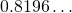, what about the exact Gaussian kernel value?Close enough. Of course, this could be done for any number of dimensions, so let us conclude the post with the new observation we made:

The features of the unnormalized-dimensional Gaussian kernel are: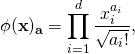where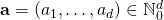.

The Gaussian kernel is just the normalized version of that, and we know that the norm to divide by is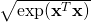. Thus we may also state the following:

The features of the-dimensional Gaussian kernel are: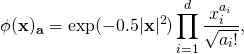where.

That's it, now you have seen the soul of the Gaussian kernel.

Posted by Konstantin @ 9:09 pm

• ### 4 Comments

1. wiwat wuwong on 17.04.2017 at 08:10 (Reply)

Thank you Sir! I really appreciate your post -- eye opening to Gaussian kernels :_

2. Keping on 22.04.2017 at 04:16 (Reply)

Perfect explanation! Thank you!

3. yaswant on 19.02.2019 at 13:37 (Reply)

Simple and to the point explanation. Thank you!!!

4. Rajendra Marathe on 15.04.2019 at 05:01 (Reply)

Nice explaination!
I have been struggling with the concept for a long time
Thank you , Sir!

Please note: Comment moderation is enabled and may delay your comment. There is no need to resubmit your comment.

### Calendar

November 2016
M T W T F S S
« Jul   Mar »
123456
78910111213
14151617181920
21222324252627
282930